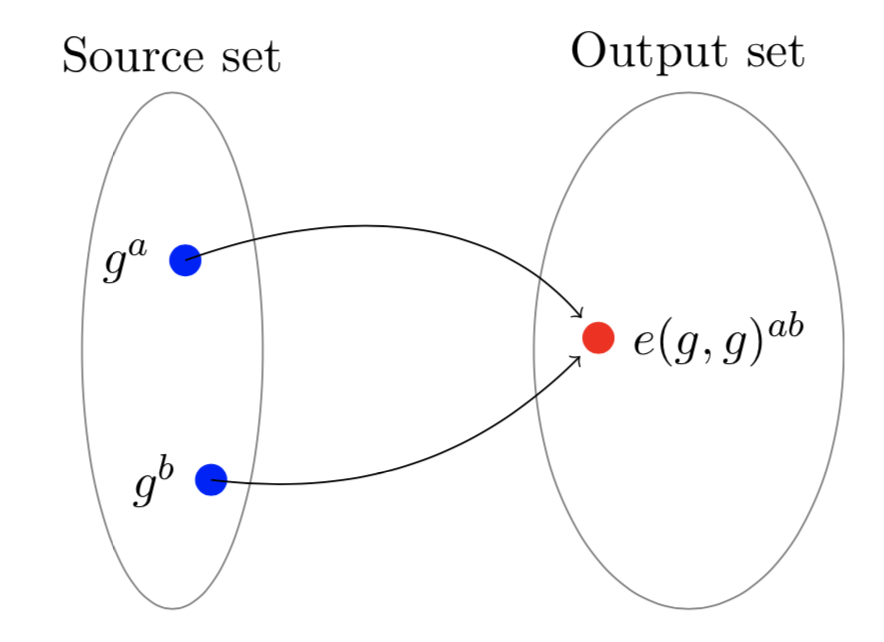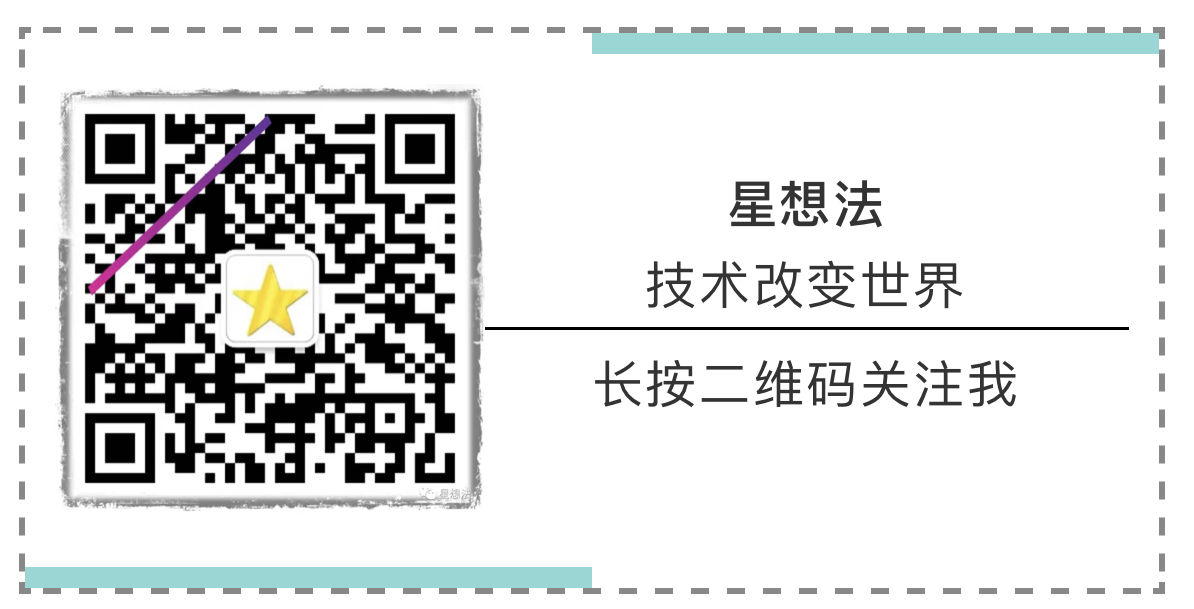• Zcash是这样用零知识证明零知识证明中的超级新星：zk-SNARKs V神讲解zk-SNARKs内部原理（上） V神讲解zk-SNARKs内部原理（下） （李康）区块链算法：零知识证明算法之zkSNARKs... 零知识证明 - Trapdoor团队...
一些资料:

Zcash是这样用零知识证明的

零知识证明中的超级新星：zk-SNARKs

V神讲解zk-SNARKs内部原理（上）

V神讲解zk-SNARKs内部原理（下）

（李康）区块链算法：零知识证明算法之zkSNARKs...

零知识证明 - Trapdoor团队发现PoREP严重漏洞

印钞机的秘密：USDT增发最全技术细节首次披露

展开全文• 零知识证明是什么：零知识证明是指证明者能够在不向验证者提供信息本身内容的情况下使验证者相信某一论断是真实可信的一种技术。举例：A向B证明自己拥有某个房间的钥匙（假设该房间只能用钥匙打开锁），这时候A可以...
• 理解为什么以及如何基于多项式构造零知识证明，这篇文章讲的比较清楚。虽然文章只讲到了皮诺曹协议，但是足够理解基于多项式构造零知识证明的本质。想深入零知识证明的小伙伴都建议看看。 ...以下是我对这篇文章的理解...
理解为什么以及如何基于多项式构造零知识证明，这篇文章讲的比较清楚。虽然文章只讲到了皮诺曹协议，但是足够理解基于多项式构造零知识证明的本质。想深入零知识证明的小伙伴都建议看看。
http://petkus.info/papers/WhyAndHowZkSnarkWorks.pdf
以下是我对这篇文章的理解和总结。原文由浅入深地从一个个简单版本，慢慢推导出实用的证明协议。
协议0 - 直观版本
随机抽查，随机提供样本，对照结果。这种协议，需要通过设定随机抽查的次数，确定安全系数。
协议1 - 从多项式的系数证明开始
最简单的d阶多项式，可以随机选择一个点x，让prover通过多项式计算生成y，verifier可以查看y是否正确。不同的多项式，最多有d个相交的点（相等的点）。
如果x/y的取值范围很大（设为n），在不知道原始多项式的情况下，能正确给出证明的概率比较低：d/n。多项式，能在一次交互，就能获取比较好的安全系数（如果n远大于d的话，将近100%）。比版本0，优秀不少。
这种协议还是比较简单和原始。证明建立在双方还是相互信任的基础上。本质上，prover并没有证明他/她知道多项式。证明本身并不能推出他/她知道一个确定的多项式。知道一个值，并不代表知道一个多项式。而且，如果多项式的值范围不大的话，证明者可以随机选择一个值撞概率。
协议2 - 基于多项式因式分解改进
假设一个多项式可以分解成：p(x) = t(x)h(x)。t(x)是目标多项式，是由p(x)的部分解组成的多项式：$t(x) = (x-x_0)(x-x_1) ... (x-x_{d-1})$。也就是说，证明者需要证明的一部分，证明者知道一个多项式的部分解。从验证者的角度看，既然你知道一个多项式的部分解，那你知道的多项式一定能整除t(x)。在随机挑选x的情况下，你都能给出正确的证明，即可认定你知道这个满足条件的多项式。

验证者，随机生成r，并计算出t®，将r发送给证明者

证明者，计算h(x) = p(x)/t(x)，并给出p®以及h®

验证者验证，是否p®=t®h®

该版本要求证明者，知道一个能整除t(x)的多项式。但比较容易发现，该协议存在如下的问题：
1/ 证明者，可以自己计算出t®，随机生成h®，并构造出p® = t®h®
2/ 即使不像1，证明者不考虑多项式，直接通过结果伪造证明。证明者，因为知道随机数r，证明者可以使用任何一个多项式，保证存在相同点(r, t®h®)。这样，证明的p®和h®虽然和正确的证明是一样的，但是，证明者却不需要知道正确的多项式。
3/ 证明者，可以自己构造多项式，只要满足h(x)=p(x)/t(x)即可。
版本3 - 引入同态加密
协议2中的问题1/2，都是因为证明者知道随机数r以及t®。引入同态加密解决。数据是加密的，在加密数据的基础上可以进行计算，能和原始数据计算具有同样的“形态”。
在模运算基础上的幂次计算，是同态加密的一种方式，表示为: $E(v) = g^v(mod\ n)$，其中v是需要加密的数据。在同态加密的基础上，可以定义运算：
加法：加密结果相乘，加密结果相乘等于原始数据相加后的加密结果
乘法：幂次运算，一个加密结果进行幂次计算等于两个原始数据乘法的加密结果（注意不是同态乘法）
除法：相对复杂，不深究
不要被加法（相乘），乘法（幂次）迷惑了。你可以认为，加密结果加法是通过加密结果的乘法实现的。
注意，乘法不支持两个加密结果乘法。也就是说，只支持一个原始数据和一个同态加密结果进行乘法操作。
有了同态加密，一个多项式的加密结果，可以通过多项式的各个项的加密结果计算。
比如说，一个多项式$p(x) = x^3 - 3x^2 + 2x$。p(x)的加密结果可以通过如下的方式计算：
$E(X^3)^1\cdot E(x^2)^{-3}\cdot E(x)^2 = (g^{x^3})^1 \cdot (g^{x^2})^{-3} \cdot (g^{x})^2 = g^{x^3-3x^2+2x}$
显而易见，一个多项式的值，可以通过各项的加密结果乘上各项的系数，计算出多项式的加密结果。

验证者，随机挑选s，计算出多项式各项的加密结果$E(s^0), E(s^1), ... E(s^d)$，并将这些值发给证明者。同时计算出目标多项式的加密值t(s)
证明者先计算出h(x) = p(x)/t(x)，并通过多项式加密计算的方法计算出E(p(s)) 和 E(h(s))
验证者验证：E(p(s)) = E(h(s))*t(s)是否成立

这个协议，验证者没有暴露随机值s，证明者只能通过多项式计算出h，从而计算出证明。这个协议，也有问题，证明者有可能只用验证者提供的各项加密结果中的几项来构造证明。并且，聪明的证明者可以自己通过多项式的各项的加密结果计算出t(s)。在知道t(s)的情况下，要构造一个证明非常容易，只需要满足幂次计算即可。
版本4 - KEA（Knowledge-of-Exponent Assumption)
假设Alice想获得a的幂次结果，具体的幂次不关心。为了限制幂次结果的提供者Bob只在a上进行幂次操作，Alice提供$a$以及$a^{\alpha}$。Bob选择一个幂次$c$，计算$b = a^c mod \ n$以及$b' = (a')^c mod\ n$，并发送给Alice。Alice通过检查$b^\alpha = b'$确定是否b是在a基础上的幂次计算。
$(a, a^\alpha)$就是“$\alpha$对"。通过一个“$\alpha$对"，数据进行同样的幂次操作。
在上述同态加密的情况下，幂次计算，是同态加密后的乘法操作。举个例子，有个简单的多项式$f(x)=c\cdot x$，验证者为了保证证明者在随机选择的s的加密结果上都“乘以”c，提供$(g^s, g^{\alpha\cdot s})$。证明者，通过提供$((g^{s})^{c},(g^{\alpha\cdot s})^{c})$，证明计算是对同一个加密结果进行了乘法操作。
因为同态加法成立，该方法可以从简单的多项式可以扩展到一半多项式。

验证者提供$g^{s^0}, g^{s^1}, ... , g^{s^d}$以及$g^{\alpha\cdot s^0}, g^{\alpha\cdot s^1}, ... , g^{\alpha\cdot s^d}$
证明者分别计算两组值：

$g^p = g^{p(s)} = (g^{s^0})^{c_0}\cdot (g^{s^1})^{c_1} \cdot... \cdot (g^{s^d})^{c_d}$
$g^{p'} = g^{\alpha p(s)} = (g^{\alpha s^0})^{c_0}\cdot (g^{\alpha s^1})^{c_1} \cdot ... \cdot (g^{\alpha s^d})^{c_d}$

验证者验证：$(g^p)^{\alpha} = g ^ {p'}$

从证明可以推导出：$g^{p'} = g^{c_0 \alpha s^0 + c_1 \alpha s^1+ ... + c_d \alpha s^d} = g^{\alpha(c_0 s^0 + c_1 s^1+ ... + c_d s^d)}$。
该协议很好的限制证明者必须在多项式的计算下，每个系数都正确“计算”。但是，这个协议并没有保护证明者的知识：验证者可以从证明信息中暴力反推多项式系数（毕竟现实场景下系数的组合还不算多）。
版本5 - ZK（零知识）
为了保护证明信息，也就是保护$c_0, c_1 ... c_d$信息。还是利用“偏移”，在$g^p 和 g^{p'}$都“乘”上一个随机系数$\delta$。即使验证者能枚举出$(\delta c_0, \delta c_1, \delta c_2 ... \delta c_d)$，也非常难获取$(c_0, c_1, ... c_d)$。
版本6 - 无交互
之前所有的协议都是交互式的。交互式协议有些问题：

验证者可以和证明者串通，伪造证明
验证者，自己生成证明
在整个交互过程中，验证者必须保存$\alpha$和$t(s)$。可能会造成其他攻击的可能。

配对函数和双线性映射
到目前为止，验证者需要验证如下的两个等式：
$g^p = (g^{h})^{t(s)}$
$(g^p)^\alpha = g^{p'}$
假设，验证者不存储$\alpha$和$t(s)$，而存储对应的加密数据。这样在验证的时候，$g^h$和$g^{t(s)}$两个加密结果“相乘“，$g^p$和$g^\alpha$”相乘“。从同态加密的定义，我们发现两个加密结果不能相乘。于是引入了新的计算特性：双线性映射。
$e(g^a, g^b) = e(g, g)^{ab}$
双线性映射的核心性质，可以表达成如下的等式：
$e(g^a, g^b) = e(g^b, g^a) = e(g^{ab}, g^1) = e(g^1, g^{ab}) = e(g^1, g^a)^b = e(g^1, g^1)^{ab}$值的一提的是，e配对函数也具有加法同态：
$e(g^a, g^b)\cdot e(g^c, g^d) = e(g^1, g^1)^{ab} \cdot e(g^1, g^1)^{cd} = e(g^1, g^1)^{ab+cd}$
到此，一个zk-SNARK的协议样子就出来了：

Setup

随机产生$s$和$\alpha$
计算生成$g^\alpha$以及$g^{s^i},g^{\alpha s^i} i\in d$，其中$(g^{s^i},g^{\alpha s^i} i\in d)$为生成密钥，$(g^\alpha, g^{t(s)})$为验证密钥

Prove

计算h(x) = p(x)/t(x)
利用$g^{s^i}$计算$g^{p(s)}$和$g^{h(s)}$
利用$g^{\alpha s^i}$计算$g^{\alpha p(s)}$
随机生成$\delta$
生成证明$\pi = (g^{\delta p(s)}, g^{\delta h(s)}, g^{\delta \alpha p(s)})$

Verification

假设证明$\pi = (g^p, g^h, g^{p'})$
检查等式$e(g^{p'}, g) = e(g^p, g^{\alpha})$
检查等式$e(g^p, g) = e(g^{t(s)}, g^h)$

扩展到一般计算
如果把一个算子的左右两个输入和输出，都看作多项式的话：
$l(x)\ operator\ r(x) = o(x)$
也就是说，$l(x)\ operator\ r(x) - o(x)=0$
可以把$l(x)\ operator\ r(x) - o(x)$看成一个多项式，类似上述的p(x)。通过之前的协议，可以证明证明者知道一个多项式，但是，并没有证明这个多项式的组成方式（不一定具有$l(x)\ operator\ r(x) - o(x)$的形式）。
通用版本0 - 支持乘法算子
如果这个算子是乘法的话，并且如果随机数为s的话，$l(s)\cdot r(s) - o(s) = t(s)h(s)$。也就是说，验证者除了需要验证l，r以及o是多项式外，还需要在加密空间验证等式成立。因为在加密空间做“减”法相对麻烦，需要算逆，可以将等式稍稍变形：
$l(s)\cdot r(s)= t(s)h(s) + o(s)$
这样，加密空间就能用配对函数进行验证：
$e(g^{l(s)}, g^{r(s)}) = e(g^{t(s)}, g^{h(s)})\cdot e(g^{o(s)}, g)$
$e(g,g)^{l(s)r(s)} = e(g,g)^{t(s)h(s)}\cdot e(g, g)^{o(s)}$
$e(g,g)^{l(s)r(s)} = e(g,g)^{t(s)h(s)+o(s)}$
这样，在之前的协议基础上，可以扩展为：

Prove

计算$h(x) = \frac{l(x)r(x)-o(x)}{t(x)}$
利用$g^{s^i}$计算$g^{l(s)}$，$g^{r(s)}$，$g^{o(s)}$和$g^{h(s)}$
利用$g^{\alpha s^i}$计算$g^{\alpha l(s)}$，$g^{\alpha r(s)}$和$g^{\alpha o(s)}$
生成证明$\pi = (g^{l(s)}, g^{r(s)}, g^{o(s)}, g^{h(s)},g^{\alpha l(s)}, g^{\alpha r(s)}, g^{\alpha o(s)})$

Verification

假设证明$\pi = (g^l,g^r,g^o, g^h, g^{l'},g^{r'},g^{o'})$
检查等式$e(g^{l'}, g) = e(g^l, g^{\alpha})$
检查等式$e(g^{r'}, g) = e(g^r, g^{\alpha})$
检查等式$e(g^{o'}, g) = e(g^o, g^{\alpha})$
检查等式$e(g^l, g^r) = e(g^{t(s)}, g^h)\cdot e(g^o, g)$

多个乘法，可以采用中间变量，分解成多个乘法操作。比如a*b*c，可以分解成：
$a*b = r_1$
$r_1*c=r_2$
多个乘法表达式，同样可以表达成$l(x)\cdot r(x) = o(x)$。和前一个例子不同的是，需要选择两个x，保证等式成立。可以通过差值计算出l(x), r(x)以及o(x)。通过通用版本0的协议，可以进行证明（扩展t(x) ）。
通用版本1 - 多项式$\alpha$对
之前讲的$\alpha$对，都是针对多项式中的某一个项。整个多项式，也可以实现$\alpha$ 对，保证某个多项式乘以一个系数。

Setup

随机产生$s$和$\alpha$
计算生成$g^\alpha$以及$g^{l(s)}$ 和$g^{\alpha l(s)}$

Prove

如果多项式的系数为$v$
计算生成$(g^{vl(s)}, g^{v \alpha l(s)})$

Verification

假设证明$\pi = (g^l, g^{l'})$
检查等式$e(g^{l'}, g) = e(g^l, g^{\alpha})$

这个协议有个问题，在多项式上做任何偏移，也能让验证等式成立。
$g^{vl(s)}\cdot g^{v'} = g^{vl(s)+v'}$
$g^{\alpha v l(s)} \cdot (g^{\alpha})^{v'} = g^{\alpha (vl(s) + v')}$
$e(g^{\alpha(vl(s)+v')}, g) = e(g^{vl(s)+v'}, g^{\alpha})$
在多个计算情况下，某个算子的输入可能是由多个变量组成：
$a \times b = r_1$
$a \times c = r_2$
$d \times c = r_3$
可以用如下的多项式来表达左输入：
$L(x) = al_a(x) + d l_d(x)$
$l_a(x)$在x=1，2的时候为1， x=2的时候为0。
$l_d(x)$在x=1，2的时候为0， x=2的时候为1。
$\alpha$对，不仅对单个多项式有限制作用，对多项式的组合同样有用：

Setup

随机产生$s$和$\alpha$
计算生成$g^\alpha$以及$g^{l_a(s)}, g^{l_d(s)}$ 和$g^{\alpha l_a(s)},g^{\alpha l_d(s)}$

Prove

计算生成$g^{L(s)} = g^{al_a(x) + dl_d(x)} = (g^{l_a(s)})^{a}\cdot(g^{l_d(s)})^{d}$
计算生成$g^{\alpha L(s)} = g^{\alpha al_a(x) + \alpha dl_d(x)} = (g^{\alpha l_a(s)})^{a}\cdot(g^{\alpha l_d(s)})^{d}$

Verification

假设证明$\pi = (g^L, g^{L'})$
检查等式$e(g^{L'}, g) = e(g^L, g^{\alpha})$

也就是说，$\alpha$对能保证一种计算结构，加密数据乘上系数的结构。加密数据本身具有加法同态，所以，可以从多项式的一项，扩展为多项式，再扩展为多个多项式。
通用版本2 - 通用计算
计算表达也进一步延伸，每个算子的输入和输出都可以是多个变量的累积。
$\sum_{i=1}^n c_{l,i}\cdot v_i \times \sum_{i=1}^n c_{r, i}\cdot v_i = \sum_{i=1}^{n} c_{o,i}\cdot v_i$
其中，$v_i$是各个变量（包括临时变量）。
假设存在d 个计算表达式，变量个数是n，相应的系数是：$C_{L,i,j}, C_{R,i,j}, C_{O,i,j} \ i\in {1...n}, j\in {1...d}$

Setup

通过插值，计算出$l_i(x)，r_i(x), o_i(x), i\in{1...n}$
随机产生$s$和$\alpha$
计算生成$g^\alpha, g^{s^k} k \in {1...d}$以及$g^{l_i(s)}, g^{r_i(s)}, g^{o_i(s)}$ 和$g^{\alpha l_i(s)},g^{\alpha r_i(s)},g^{\alpha o_i(s)}$

Prove

计算$h(x) = \frac{L(x)\times R(x)-O(x)}{t(x)}$，其中$L(x) = \sum_{i=1}^n v_i\cdot li(x)$，R(x)/O(x)类似
计算生成$g^{L(s)} = \prod_{i=1}^n (g^{l_i(s)})^{v_i},g^{R(s)} = \prod_{i=1}^n (g^{r_i(s)})^{v_i},g^{O(s)} = \prod_{i=1}^n (g^{o_i(s)})^{v_i}$
计算生成$g^{\alpha L(s)} = \prod_{i=1}^n (g^{\alpha l_i(s)})^{v_i},g^{\alpha R(s)} = \prod_{i=1}^n (g^{\alpha r_i(s)})^{v_i},g^{\alpha O(s)} = \prod_{i=1}^n (g^{\alpha o_i(s)})^{v_i}$
计算生成$g^{h(s)}$

Verification

假设证明$\pi = (g^L, g^{R}, g^{O}, g^{L'}, g^{R'}, g^{O'}, g^{h})$
检查等式$e(g^{L'}, g) = e(g^L, g^{\alpha}),e(g^{R'}, g) = e(g^R, g^{\alpha}),e(g^{O'}, g) = e(g^O, g^{\alpha})$
检查等式$e(g^L, g^R) = e(g^t, g^h)\cdot e(g^O, g)$

通用版本3 - 固定输入和输出
上述的协议因为对l，r，以及o使用的是同一个$\alpha$值，存在如下的问题：

L(x)的计算采用R(x)/O(x)中的多项式：$L'(s) = o_1(s) + r_1(s) + r_5(s)+ ...$

输入/输出，换位 ：$O(s) \times R(s) = L(s)$ （证明者，提供证明时，只是交换O/L的位置，同样能通过验证）

重用同样的输入: $L(s) \times L(s) = O(s)$ （证明者，提供证明时，用L(s)代替R(s) ，同样能通过验证）

解决上述问题的简单的做法，分别对L(x)/R(x)/O(x)使用不同的$\alpha$对。
通用版本4 - 限定变量
细心一点会发现，之前的协议并没有限制证明者使用“一致”的变量的取值。也就是说，证明者，可以在计算L(x), R(x), O(x)的时候，使用不同的$v_i$。
如何限制证明者使用同样的变量值？还是采用"$\alpha$对"的思想，将多项式“累积”在一起，先用统一一个偏移进行限制。
$e(g^{v_{L,i}\cdot l_i(s)}\cdot g^{v_{R,i} \cdot r_i(s)}\cdot g^{v_{O,i}\cdot o_i(s)}, g^\beta) = e (g^{v_{\beta, i}\cdot \beta (l_i(s)+r_i(s)+o_i(s))}, g)$
如果$v_{L,i}=v_{R,i}=v_{O,i}=v_{\beta, i}$的话，显然表达式成立。但是，在一些场景下，比如证明者知道L(x)=R(x)的情况下，不需要变量相等，等式也能成立。
$(V_{L,i}\cdot l_i(s) + V_{R,i}\cdot r_i(s) + V_{O,i}\cdot o_i(s))\cdot \beta = V_{\beta, i}\cdot \beta \cdot (l_i(s) + r_i(s) + o_i(s))$
假设$w=l(s)=r(s)$，并且$y=o(s)$：
$\beta(v_L w+ v_R w + v_O y) = v_{\beta}\cdot \beta(w+w+y)$
证明者，知道这些信息，要让等式成立，可以让$v_{\beta} = v_{O}, v_{L} = 2v_O - v_R$。也就是存在可能性，不同的变量值，验证等式依然成立。
进一步优化验证等式，让每个变量采用不同的偏移，记为$\beta$对：

Setup

额外随机生成$\beta_l, \beta_r, \beta_o$
额外计算$\{g^{\beta_l l_i(s)+\beta_r r_i(s)+\beta_o o_i(s)}\} i\in \{1...n\}$

Prove

额外计算$g^{Z(s)} = \sum_{i=1}^{n}g^{z_i(s)} = g^{\beta_l L(s) + \beta_r R(s) + \beta_o O(s)}$

Verification

验证等式：$e(g^L, g^{\beta_l})\cdot e(g^R, g^{\beta_r}) \cdot e(g^O, g^{\beta_o}) = e(g^Z, g)$

因为:
$L\cdot \beta_l + R\cdot \beta_r + O\cdot \beta_o = \sum_{i=0}^n v_il_i(s)\beta_l + v_i r_i(s) \beta_r + v_i o_i(s) \beta_o$
$= \sum_{i=0}^n v_i(l_i(s)\beta_l + r_i(s) \beta_r + o_i(s) \beta_o)$
既然，L/R/O采用通用的变量值v，则L+R+O，可以看成合并的一个多项式。为了避免证明者利用L/R/O的关系，伪造证明，使用多个$\beta$对。
也就是说，$\alpha$对限制计算是按照指定的多项式乘加，$\beta$对限制计算采用同样的变量值。
通用版本5 - 不可变形
上述的协议还存在两种变形问题：
1/ 变量的多项式变形：$\alpha$对限制了计算是按照指定的多项式乘加。比如说，L是多个$l_i(x)$乘加。但是因为证明者知道$g^{l_i(x)}$和$g^{\alpha}$，可以从验证者提供的信息构造$g^{l_i(x)+1}$。
2/ 变量取值变形：$\beta$对虽然限制了计算需要是$l_i(x) + r_i(x) + o_i(x)$的形式，但并没有限制在证明数据上同时偏移。
这些变形的问题，因为证明者完全知道$\beta$的加密结果。解决的办法是：引入$\gamma$，进一步隐藏$\beta$的加密结果。

Setup

额外随机生成$\beta_l, \beta_r, \beta_o, \gamma$
额外设置验证密钥：$g^{\beta_l \gamma},g^{\beta_r \gamma},g^{\beta_o \gamma},g^{\gamma}$

Prove

额外计算$g^{Z(s)} = \sum_{i=1}^{n}g^{z_i(s)} = g^{\beta_l \gamma L(s) + \beta_r \gamma R(s) + \beta_o \gamma O(s)}$

Verification

验证等式：$e(g^L, g^{\beta_l \gamma})\cdot e(g^R, g^{\beta_r \gamma}) \cdot e(g^O, g^{\beta_o \gamma}) = e(g^Z, g^{\gamma})$

通用版本6 - 优化配对计算个数
上一个协议需要4个配对函数的计算。皮诺曹协议（Pinocchio protocol ）通过对L/R/O，使用不同的生成元，减少了配对函数的计算。

Setup

随机生成$\alpha_l,\alpha_r, \alpha_o, \beta, \gamma，\rho_l, \rho_r$，并设置$\rho_o = \rho_l \cdot \rho_r$
生成三个生成元：$g_l=g^{\rho_l}, g_r=g^{\rho_r}, g_o=g^{\rho_o}$
设置证明密钥：$\{g^{s^k}\}_{k\in d}, \{g_l^{l_i(s)},g_r^{r_i(s)}, g_o^{o_i(s)}, g_l^{\alpha_l l_i(s)},g_r^{\alpha_r r_i(s)}, g_o^{\alpha_o o_i(s)},g_l^{\beta l_i(s)},g_r^{\beta r_i(s)}, g_o^{\beta o_i(s)}\}$
设置验证密钥：$g_o^{t(s)}, g^{a_l}, g^{a_r}, g^{a_o}, g^{\beta \gamma},g^{\gamma}$

Prove

额外计算$g^{Z(s)} = \prod_{i=1}^n(g_l^{\beta l_i(s)}\cdot g_r^{\beta r_i(s)}\cdot g_o^{\beta o_i(s)})^{v_i}$

Verification

验证是否采用指定的多项式：$e(g_l^{L'},g) = e(g_l^L, g^{\alpha_l})$，同样检查R和O
验证是否采用一致的变量值：$e(g_l^L \cdot g_r^R \cdot g_o^O, g^{\beta \gamma}) = e(g^Z, g^{\gamma})$
验证计算是否成立：$e(g_l^L, g_r^R) = e(g_o^t, g^h)\cdot e(g_o^O, g)$

在理解了皮诺曹协议的基础上，再看Groth16算法，就比较简单了。
总结：

协议都是建立在多项式基础上。
多项式分解，提供了一个能证明证明者知道一个满足一定条件多项式的方法。为了能让这种方法工作需要其他一些条件：

同态加密：随机数以及对应多项式的各个项都能加密的同时，还能进行乘法和加法的计算。同态加密的作用是防止证明者能反推随机数，直接伪造证明。同时还能在加密数据的基础上提供证明者需要的计算。
"$\alpha$对"保证证明者的计算都是在加密数据乘法和加法的基础。也就是说，证明者的计算是基于多项式的。
双线性映射，能让两个加密数据映射成原始数据乘积的加密结果。

zk的实现反倒简单。在证明数据上进行一个偏移。保证证明者的原始数据不泄漏。
Trusted Setup生成初始的参数。
$\alpha$参数限制多项式形式。$\beta$参数保证多个多项式采用同样的系数。$\gamma$参数防止多项式偏移。展开全文• 区块链是一种以密码学算法为基础的点对点分布式账本技术,然而,...总结了已有的隐私保护方案,重点聚焦于零知识证明技术,阐述并分析了零知识证明应用到区块链隐私保护方案中的技术挑战,并给出了具有指导意义的解决方案。区块链
• 那么对于一些隐私和安全性要求比较高的传统企业，必然会提出在实现公开信任和自动交易的基础上提供商用级隐私保护的诉求，那么对于看似矛盾的倡导公开透明与商用级隐私保护这个难题，零知识证明技术就是一个最佳的...
缘起
进来参与了很多安永的区块链技术活动，安永发布了基于零知识证明的Nightfall框架。因此决定把零知识证明这个加密体系中的硬核知识给全方位的梳理一遍，就有了此零知识证明系列文章。
零知识证明的一则小故事
零知识证明（也叫做最小泄露证明系统）一般来说分为两大体系，一个是交互式零知识证明体系和非交互式零知识证明体系。下列的这则小故事是网络上盛传的交互式零知识证明故事，详细故事如下：
很久很久以前，有一个藏着财宝的山洞，阿里巴巴因为知道开启山洞石门的咒语，而被强盗抓住。如果说出咒语，失去利用价值也是一死；如果坚持不说，强盗也会杀死他。
两难的阿里巴巴却想了一个好办法，他让强盗随机下指令：“你们只需离我一箭之遥，用弓箭指着我，你们举起右手我就念咒语打开石门，举起左手我就念咒语关上石门，如果我做不到或伺机逃跑，你们就用弓箭射死我。”
重复多次后，强盗始终无法获知咒语，却可以相信阿里巴巴拥有咒语的事实，阿里巴巴也保住了性命。
那么你可能想知道交互式零知识证明和非交互式零知识证明有什么区别？通过上述故事可以知道，交互式零知识证明需要证明人P和验证者V多次信息交互验证，这样会带来时间和成本的耗损。如果是一个交易体系中的验证模块，那么如此频繁的交互势必让整个系统带来觉得时间和成本损失。
那么非交互式零知识证明对比交互式零知识证明的异同点在哪呢？那么我们需要了解零知识证明体系的特性。
初识零知识证明
零知识证明系统：零知识证明系统包括两部分：宣称某一命题为真的示证者P(prover)和确认该命题确实为真的验证者V(verifier)。证明是通过这两部分之间的交互来执行的。在零知识协议的结尾，验证者只有当命题为真时才会确认。但是，如果示证者宣称一个错误的命题，那么验证者完全可能发现这个错误。
零知识证明协议：零知识证明(Zero—Knowledge Proof)起源于最小泄露证明。设P表示掌握某些信息，并希望证实这一事实的实体，设V是证明这一事实的实体。假如某个协议向V证明P的确掌握某些信息，但V无法推断出这些信息是什么，我们称P实现了最小泄露证明。不仅如此，如果V除了知道P能够证明某一事实外，不能够得到其他任何知识，我们称P实现了零知识证明，相应的协议称作零知识协议。
零知识证明性质：通过上述的零知识证明系统和协议我们可以知道，零知识证明有如下三个性质：
(1)正确性。P无法欺骗V。换言之，若P不知道一个定理的证明方法，则P使V相信他会证明定理的概率很低。
(2)完备性。V无法欺骗P。若P知道一个定理的证明方法，则P使V以绝对优势的概率相信他能证明。
(3)零知识性。V无法获取任何额外的知识。
零知识证明属性：同时考虑到示证者P可能存在欺骗的行为，因此零知识证明满足如下三个属性：
(1)如果语句为真，诚实的验证者（即：正确遵循协议的验证者）将由诚实的证明者确信这一事实。
(2)如果语句为假，不排除有概率欺骗者可以说服诚实的验证者它是真的。
(3)如果语句为真，证明者的目的就是向验证者证明并使验证者相信自己知道或拥有某一消息，而在证明过程中不可向验证者泄漏任何有关被证明消息的内容。
因此，零知识证明并不是数学意义上的证明，因为它存在小概率的误差，欺骗者有可能通过虚假陈述骗过证明者。换句话来说，零知识证明是概率证明而不是确定性证明。但是也存在有技术能将误差降低到可以忽略的值。
综上，我们可以看到，拥有上述四个特征的零知识证明可以用来对具体场景的应用：
1、A要向B证明自己拥有某个房间的钥匙，假设该房间只能用钥匙打开锁，而其他任何方法都打不开时，零知识证明的应用可以是B确定该房间内有某一物体，A用自己拥有的钥匙打开该房间的门，然后把物体拿出来出示给B，从而证明自己确实拥有该房间的钥匙。
该场景属于零知识证明。它的好处在于，在整个证明的过程中，B始终不能看到钥匙的样子，从而避免了钥匙的泄露。
2、A拥有B的公钥，A没有见过B，而B见过A的照片，偶然一天两个人见面了，B认出了A，但A不能确定面前的人是否是B，这时B要向A证明自己是B时，零知识证明的应用可以是A给出一个随机值，并使用B的公钥对其加密，然后将加密后的数据交给B，B用自己的私钥解密并展示给A，如果与A给出的随机值相同，则证明对方是B。
该场景同样属于零知识证明。它的好处在于，在整个证明的过程中，双方私钥信息不公式，不存在泄漏的安全风险。
3、有一个缺口环形的长廊，出口和入口距离非常近（在目距之内），但走廊中间某处有一道只能用钥匙打开的门，A要向B证明自己拥有该门的钥匙。采用零知识证明，则B看着A从入口进入走廊，然后又从出口走出走廊，这时B没有得到任何关于这个钥匙的信息，但是完全可以证明A拥有钥匙。
该场景也属于零知识证明。它的是基于已知的结果信息进行对比，通过结果来反推条件的一致性。
零知识证明与区块链
对区块链有了解的同学肯定会知道区块链的如下几个特征：
区块链的五大特点
1、去中心化——无需第三方介入，实现人与人点对点交易和互动。
2、不可篡改性——数据信息一旦被写入区块中就不能更改撤销。
3、开放性——区块链的系统数据是公开透明的，每个人都可以参与进来。
4、匿名性——区块链上面没有个人的信息，因为这些都是加密的，是一堆数字字母组成的字符串。
5、分布式自治——区块链采用基于协商一致的规范和协议（基于一套公开透明的共识算法），然后各个节点就按照这个规范来操作，这样就是所有的东西都有机器完成。
因此一个公开透明去中心化的开放区块链体系如果要进行商业化运作，那么对于一些隐私和安全性要求比较高的传统企业，必然会提出在实现公开信任和自动交易的基础上提供商用级隐私保护的诉求，那么对于看似矛盾的倡导公开透明与商用级隐私保护这个难题，零知识证明技术就是一个最佳的解决方案。
零知识证明技术应用在区块链上，可以轻易的实现在不泄漏交易双方的隐私信息同时，又能让示证者P证明自己拥有某种信息或者链上资产，最终让双方在互信和公开透明的区块链网络上完成既定的交易。
在下一讲，我们会详细分析zk-SNARK这个已经实现了的区块链零知识证明协议。


展开全文区块链
• 我们可以自由的以这些术语给朋克乐队或Tumbirs博客起名字，像是“硬核谓词(hard-core predicate)”、“陷门函数(trapdoor function)”，或“不可差分密码分析(impossible differential cryptanalysis)”等热词都受到...
当今密码学世界中最酷炫的一件事，莫过于那些优美又神秘的专有名词。我们可以自由的以这些术语给朋克乐队或 Tumbirs 博客起名字，像是“硬核谓词(hard-core predicate)”、“陷门函数(trapdoor function)”，或“不可差分密码分析(impossible differential cryptanalysis)”等热词都受到追捧。 当然，我今天要提到的这个术语热度绝不亚于前面三者，它就是“零知识(zero knowledge)”。

事实上太过受欢迎也不一定是件好事，因为”零知识“概念如此吸引眼球，也导致许多理解错误和误用。许多人将零知识和“非常非常安全”划上等号，并将它与加密系统或匿名网络挂钩——但这是不正确的，这与真正的零知识协议毫无关系。

这一切都说明 “零知识证明” 是密码学家所设计出来最强大的工具之一，同时理解的人也相对较少。接下来，我将试着（尽可能）以 非数学 领域的表述方式，介绍什么是“零知识证明”，并解释到底是什么使得它如此特别。同时在此篇和下篇文章中，我们会谈及一些常用的零知识证明协议。

零知识起源

“零知识”的概念最早在80年代由麻省理工学院的研究人员 Shafi Goldwasser，Silvio Micali 和 Charles Rackoff 所提出。当时这些人正在研究与交互证明系统相关的问题——即一种理论系统，使得甲方（证明者）可以和乙方（验证者）交换信息，并借此说服乙方接受（通过验证）某个数学论述为真 [作者注1]。

在Goldwasser等人之前，这个领域的研究工作主要聚焦在加强证明系统的可靠性（Soundness）。也就是说原先大家都假设，会有恶意的证明者试图耍手段，误导验证者接受错误的论述。但 Goldwasser 等人却从另一个角度思考了这个问题：如果我们压根就不相信 验证者，该怎么办？

更具体的来说，他们更关心信息泄露的问题。他们抛出了这样的思考：“在验证者的验证过程中，究竟会获取多少单纯验证论述真假无需知道的额外信息。”

这里要强调一下，这个问题不是单纯的理论思考，而是在真实、具体的应用中，会面到临的问题。

我们举个例子，假设今天在真实世界有个客户端想要使用密码登录web服务器，在“真实世界”的标准操作流程中，包含将密码以哈希形式储存在客户端。我们可以将”登录“这个动作视作某种证明——也就是我们要能够提供一串输入，这串输入经过哈希运算后的值与密码的哈希相同（因为哈希运算的单向性，这串输入只能是我们的密码）。但这有个问题是：客户端实际上 知道 我们的密码。

大多数系统以这种绝对最糟糕的方式进行“证明”——客户端直接将原始密码发送给服务器，服务器重新计算密码哈希并将其与存储值进行比较。这里的问题很明显：在协议结束时，服务器已经取得我们的明文密码。 因此，保障现代密码安全很大程度上要祈祷服务器不会遭受攻击。

Goldwasser，Micali 和 Rackoff 等人提出了一种全新的方法来完成“证明”。如果零知识证明真的可行，它将允许我们在证明某些数学陈述为真的同时，保证 不会有任何不相关的信息 被透露出去。

一个“真实世界中”的案例

目前为止，我们的讨论还比较抽象。为了让大家能有更具体的概念，现在我们举个“真正的”（脑洞微开的）零知识证明例子。

请大家配合我想象一下，现在我是个电信业巨头，并且打算部署一个新的蜂窝电信网络。这个网络架构图如下（图一）。图中的每个顶点代表一个无线电塔，每一条连线（边）代表无线电塔信号 两两重叠 的区域，这意味着连线上的信号会互相干扰。这种重叠的情况是有问题的，这表示来自相邻电塔的信号会互相混淆。幸好在设计之初我预见这个问题，现在通信网络允许传递三种波段的信号，这样就避免了临近电塔信号干涉的问题。

不过现在我们有了新的挑战！这个挑战来自我该如何部署不同的波段，使得相邻的每两个电塔不具有相同波段。我们现在用不同颜色来表示不同波段，可以很快找到一种解决方案如下图二所示：当然，很多人可能已经发现我刚才是在讲述著名的算法问题——三色问题(graph three-coloring)。大家也就知道，这个问题有趣的地方在：某些非常庞大的网路中，我们很难找到解，甚至连证明问题 有解 都办不到。事实上三色问题——特别是指这种给定图形是否有解的决策问题，已经被归类为NP完全问题。

如果只是上面给的这种示例图，我们用手就能轻松找出解。但如果今天我的无线通信网路规模特别复杂而庞大，我以我所能调配的计算资源都无法找到解答的情况下，我该怎么办？我还可以把这个问题 外包 给拥有更庞大算力的人呀！比如我会去找我在谷歌的朋友帮忙。

但这又会导致另一个问题。

假设谷歌动用了大量的算力来帮我找寻有效的着色方法。当然，在我确实得到有效着色方法之前，我是不打算付钱给谷歌的。同样对谷歌来说，在我付款之前，谷歌也不愿意给我着色方法的副本。因此我俩就会陷入僵局。

在现实生活中，可能有点常识都能解决这个困境，但这涉及律师和账户托管。不过今天这篇博客不是表述现实生活，而是关于密码学的。如果你曾经看过任何加密相关文章，你可能知道，解决这种困境的正确方法，就是 想出一个绝对疯狂的技术手段 ！

一种疯狂的技术手段（用帽子！）

谷歌的工程师向Micali 等人在麻省理工进行了咨询。他们想出了一种非常聪明而优雅，甚至不需要任何计算机的方法来打破上述的僵局。我们只需要一个大仓库、大量的蜡笔和纸张。噢，对了，我们还需要一堆的帽子！[作者注2]

下面是运作原理。

首先，我先进入仓库，在地板上铺满纸张，并在空白的纸上画出电塔图。接下来我离开仓库，换谷歌工程师进入仓库。谷歌工程师先从一大堆的蜡笔中，随机选定三个颜色（与上面的例子一样，我们假设随机选中红色/蓝色/紫色），并在纸上照着他们的解决方案上色。请注意，用哪种颜色上色并不重要，只要上色的方案是有效的就行！

谷歌工程师们上色结束后，在离开仓库前，他们会先用帽子把每个纸上的电塔盖住。所以当我回到仓库的时候，我会看到如下图三：显然的，这种方法保障了谷歌着色方法的秘密性。但这样对我一点帮助都没有！我不知道他们是不是进行了有效着色，或是他们根本没有着色？

为了消除我的疑虑，谷歌工程师们决定给我机会“挑战”他们的着色方案。我被允许——随机选择图上的一条边（两个相邻帽子中间的一条线），然后要求谷歌工程师揭开两边覆盖着的帽子，让我看到他们着色方案中的一小部分，如图四：产生两种结果：

如果两个点颜色相同（或是根本没有被着色！），我就可以确信谷歌工程师们对我撒谎。因此我也很清楚我不需要付钱。
如果两个点颜色不同，那么谷歌工程师 可能没有 撒谎。
第一种情况很单纯（我不付钱！），第二种情况下我要进行更多考虑。即使我刚才进行了一轮观察，毕竟我只揭开两顶帽子，只看到两个点，仍然不能保证谷歌工程师给我的方法是有效的。假如图上有 E 条不同边，在目前条件下谷歌仍有很大的可能是给了我一个无效的着色方案。实际上，在经过一次揭帽观察后，我仍有高达 (E-1)/E 的概率会被骗（假如有1000条边，有99.9%的概率这个方案无效。）

好在谷歌决定让我们再一次、重新进行观察！

我再次走出仓库，他们重新铺上新的纸张，并把空白的电塔图画上。谷歌工程师再次从大量蜡笔中随机选出三种颜色进行着色。他们再次完成有效着色方案，但使用新的三种随机颜色。

接着帽子又被盖上啦。我走进仓库再一次进行“挑战”，选择一条新的、随机的边。上述逻辑再一次适用。不过这次情况稍好，我会对谷歌工程师们多了那么一点点信心，相信他们没有对我撒谎。因为如果他们要欺骗我，他们必须连续两次都这么幸运。这当然有可能发生——但发生的可能性相对较低。现在谷歌连续两次都骗到我的概率为 [(E-1)/E] * [(E-1)/E]（在1000条边的情况下，大约有99.8%的可能性，还是很高）。

不过不用担心，我们不只可以进行两次挑战。事实上，我们可以不断的重复上述的挑战，直到我们相信谷歌给出了有效的着色方案。

切记不要盲目信我的话。感谢 Javascript，你可以通过简单的代码自己验证上面的逻辑。提醒下，我永远无法完全相信谷歌工程师是诚实的——我被骗的概率总会存在，即使概率很小。但经过大量的迭代后（E^2），我最终可以将信心提升到一个程度，那时候谷歌只剩下微不足道的概率可能骗到我——这概率低到我可以安全地把钱交给谷歌。

而且你需要知道的是，在这个过程中谷歌同样受到保护。即使我试图在挑战的过程中推敲出他们正确的着色方案，那也不要紧。因为谷歌在每一次迭代前随机更换三种新的颜色，这让我的小手段失效了。我获得的讯息对我毫无帮助，每次挑战的结果也无法被串联起来。

是什么让它“零知识”?

我对你声称，这种挑战不会泄露任何关于谷歌着色方案的信息，但请你们不要这么轻易放过我！现代密码学第一条守则就是：永远不要在未经证明的情况下相信一个人的宣称。

Goldwasser, Micali 和 Rackoff提出三个零知识证明的特性，任何零知识都必须满足。简单来说：

完整性(Completeness)。如果谷歌说的真话，那么他们最终能说服我（至少让我相信可能性非常高）。
安全性(Soundness)。只有当他们说的是真话时，谷歌才有可能说服我。
零知识性(Zero-knowledgeness)（没错，就这么叫）。我无法从中习得任何关于谷歌解决方案的信息。
我们已经讨论了完整性的论点。只要重复足够多次挑战，这个协议最终能够说服我（伴随极低的失误可能）；安全性也很容易说明，因为如果谷歌试图欺骗我，会有很大的概率会被我发现。

最难说明的就是“零知识性”。为此，我们必须进行一项非常奇怪的思想试验。

思想试验（时光机？）

我们要从一个疯狂的假设开始。假如谷歌工程师并没有大家想象中厉害，他们花了数周时间，仍然没有想出着色问题的解决办法。而现在只剩下12小时他们就得展示了！他们已经感受到了绝望。绝望使人疯狂，他们决定 诱导 我相信他们已经完成有效的着色，实际上他们并没有完成。

虽然使用时光机获得更多工作时间的想法已经不可行，但这有限的功能已经足够欺骗我。-我不知道这里到底发生了什么，但看起来好厉害的样子-

这个计划要命的简单！因为谷歌工程师们 实际上不知道 正确的着色方案，他们只能直接从大量蜡笔中随机选出颜色来涂，然后盖上帽子。如果他们足够幸运，我在挑战时选中不同颜色点，他们就可以松口气，然后继续进行挑战，以此类推。

然而不可避免的，我总会在某次挑战时揭开两顶帽子，然后发现两个相同 颜色的点！如果按照正常挑战规则，谷歌工程师们就原地崩溃了，而这也是时光机派上用场的时候。任何时候谷歌工程师们发现自己身处尴尬的情况（被我找到同色点），他们可以轻松挽回颓势。只要其中一个工程师按下时光机按钮，让时间倒退大约四分钟，然后他们再以新的完全随机方式着色。接着时间正常前进，挑战将继续进行。

实际上，时光机让谷歌工程师可以挽回在欺骗过程中的任何失误，同时让我误以为这个挑战过程完全符合规则。从谷歌工程师的角度来看，造假被挑战出来的情况只有1/3，所以整个挑战时间只会比诚实情况下（他们有有效解答）的挑战时间稍微长一点；从我的角度来看，我只会认为这是完全公平的挑战，因为我不知道时光机的存在。

最后一点，也是最重要的一点。在我的眼中，因为我压根不知道有时光机存在，所以我看到的每一次挑战结果，我都会认定这就是真实的！而统计结果也完全一致。值得再呼吁一次，在时光机作弊的情境下，谷歌工程师们绝对不知道正确着色方案。

我到底想说什么？

请注意，我们刚才举得是一个计算机仿真 的例子。在现实世界中，时间当然不能倒退，也没有人能用时光机器骗我，所以基于帽子的挑战协议是合理且可靠的。这表示在 E^2 轮挑战后，我应该相信盖着的图是被正确着色的，同时谷歌工程师们也遵守协议规则。

方才我们展示的是，如果时间不只能够前进——特别的是谷歌能“倒退”我的时间，那即使他们没有正确的着色方案，他们仍然能使挑战正常进行。

从我的角度出发，这两种情况有什么区别？当我们考虑从这两种情况下的统计分布，会发现根本没有区别，两者都表达了相同量级的有效信息。

信不信，这恰好证明了一件非常重要的事情！

具体来讲，假设我（验证者）在正常挑战协议过程中，有办法“提取”关于谷歌正确着色方案相关信息。那么当我被时光机糊弄的时候，我的“提取”策略应该仍然有效。但从我的角度来看，协议运行结果在统计学上毫无二致，我根本无法区别。

因此如果我在“公平的挑战”和“时光机实验”下，所能得到的信息量相同；且谷歌在“时光机实验”中投入的信息量为零，则证明即使在公平的挑战下，也不会透露任何相关信息给验证者知道。

又或是这恰好说明计算机科学家有时光机？？是的，我们有。（请务必保密......）

抛开帽子（也抛开时光机）

当然在现实世界我们不会想用帽子来进行协议，谷歌（可能）也没有真正意义上的时光机。

为了将整件事情串起来，我们先把这个协议放到数字世界。这需要我们构建一个相当于“帽子”功能的等价物——它既能隐藏数字价值，又能同时“绑定”（或“承诺”）创建者，这使得事实被公布后他也不能不认账。

幸运的是，我们恰好有这种完美的工具。这就是所谓数字承诺方案。这个方案允许一方在保密的情况下“承诺”给出的信息，然后再“公开”承诺的信息。这种承诺可以有很多结构组成，包含（强）加密哈希函数。[作者注3]

我们现在有了承诺方案，也就有一切电子化运行零知识证明的要素。首先证明者(Prover)可以将每个点以数字信息形式”着色“（例如以数字0,1,2），然后为每个数字信息生成数字承诺。这些数字承诺会发送给验证者(Verifier)，当验证者进行挑战的时候，证明者只需要展示对应的两个点的承诺值就行。

所以我们已经设法消除帽子了。但如何证明这个过程是零知识的？

我们现在身处数字世界，不再需要一台时光机证明与此相关的事。其中的关键在于数字世界中，零知识证明协议不是在两个人 之间运行的，而是在两方不同的计算机程序 上运行的（或是更规范地说，是概率图灵机）。

我们现在可以证明下面的定理：如果你能做出一套程序，使得验证者（计算机）能够在挑战过程中”提取“额外的有用信息，则我们就有办法在程序中加入“时光机”的功能，使得它能够在证明者没有投入任何信息的情况下（译者注：即谷歌工程师没有正确解），从“假”的挑战过程获得等量的额外信息。

因为我们现在讨论的是计算机程序，回退、回滚等倒退时间的操作根本不是难事。实际上，我们在日常使用上就不断在回滚程序。比如带有快照功能的虚拟机软件。-通过虚拟机快照进行回滚的例子示意图。一台初始虚拟机继续执行，回滚到一个初始的快照中，然后分叉到另一条新路径中执行-

即使你没有复杂的虚拟机软件，任何计算机程序也都可以回滚到先前状态。我们只需要重新启动程序，并提供完全相同的输入即可。只要输入的所有参数（包含随机数）都是相同的，程序将永远按照相同的执行路径操作。这意味着我们可以从头开始运行程序，并在需要的时间点进行“分叉(forking)”。

最终我们得到以下定理。如果存在任何的验证者计算机(Verifier)程序，它可以通过与证明者的协议交互过程中提取信息，那么证明者计算机(Prover)同样可以通过程序回滚来”欺骗“验证者——即证明者无法通过挑战，却以回滚的方式作弊。我们已经在上面给出了相同的逻辑：如果验证者程序能从真实的协议中提取信息，那么它也应该能从模拟的、会回滚的协议中获取等量的信息。又因为模拟的协议根本没有放入有效信息，因此没有可提取的信息。所以验证者计算机能提取的信息一定始终为零。

这，到底在说什么？

让我们回顾一下。根据我们上面的分析，可以知道这个协议是完整且可靠的。该可靠性的论点在我们知道没有人玩弄时间的前提下都是站得住脚的。也就是说，只要验证者计算机正常运行，并且保证没有人在进行回滚作弊的话，协议是完整且可靠的。

同时我们也证明这种协议是零知识的。我们已经证明了任何能成功提取信息的验证者程序，也一定能从回滚的协议运行中提取信息，而后者是没有信息放入的。这明显自相矛盾，间接论证该协议在任何情况下都不会泄露信息。

这一切有个重要的好处。比如在谷歌工程师向我证明他们有正确的着色方案后，我也无法将这个证明过程转传给其他人用以证明同样的事（如，法官），这使得伪造协议证明变成了不可能的事。因为法官也不能保证我们的视频是真是假，不能保证我们没有使用时光机不断回滚修改 证明。所以零知识证明只有在我们自己参与的情况下才有意义，同时我们可以确定这是实时发生的。

证明所有的NP问题！

如果你能坚持看到这儿，我敢打包票你已经准备好迎接一个大新闻！就是我们讲了半天的三色电信网络网络，其实并不有趣——至少，本身不咋地。

真正有意思的地方在于，三色问题属于NP完全问题。简单来说，这件事的奇妙之处在于任何其他的NP问题都可以转化为这个问题的实例。在一次不经意的尝试下，Goldreich, Micali 和 Wigderson 发现“有效的”零知识证明大量存在于这类问题的表述中。其中许多问题比分配蜂窝网格问题有趣得多。你只需要在NP问题中找到想要证明的论述，比如上面的哈希函数示例，然后转化为三色问题。然后再进行数字版的帽子协议就行啦！

总结

当然，单纯为了兴趣来运行这项协议，对任何人来说都是疯狂而近乎愚蠢的——因为这样做的成本包含原始状态和证人的规模大小、转化为图形的花费，以及理论上我们必须运行E^2 次才能说服某人这是有效的。

理论上说这个协议是“高效率的”，因为证明的总成本会是输入状态的多项式，但其实不然。

所以我们迄今展示的，是要表达这种证明是“可能的”。接下来我们仍然需要找到更多的实例来支撑零知识证明的可用性。

在上一篇中我们描述了任何零知识证明都必须满足的三个关键属性：

完整性（completeness）：如果证明者是诚实的，那么她最终会说服验证者。
可靠性（Soundness）：证明者只能说服验证者该陈述是否属实。
零知识性（Zero-knowledgeness）：除了知道陈述是真实的，验证者不知道任何额外的信息。
真正的挑战来自如何定义最后一个属性。 你如何判断验证者无法获取除了陈述之外的任何信息呢？

如果您没有读过前一篇文章，我可以告诉你一个由 Goldwasser，Micali和Rackoff 三人提出一个非常赞的方案。 他们认为一个协议如果满足对于每一个可能的验证者，可以证明一个叫“模拟器”的算法的存在，并且这个算法有一些非常特殊的属性，就认为它满足零知识证明 。

机械地来看，模拟器就像一个特殊的证明者。 不过与真正的证明者不同，后者以一些能够证明陈述真实性的特定信息开始， 模拟器则不会 [作者注1]。然而模拟器必须能够“欺骗”每一个验证者使他们相信该陈述是真实的，同时产生与真实证明者在统计意义学上相同（或者说无法区分）的输出结果副本。

逻辑流程非常清晰：由于模拟器没有“知识”能被提取，因此显然验证者在与之交互后无法获得任何有价值的信息。 此外，如果交互的信息副本与使用正常证明者运行的真实协议的分布相同，那么验证者对于真正的证明者的验证结果不会比对于模拟器的验证结果更精确。 （如果更精确，那么这意味着模拟器与真正的证明者的分布在统计学上是不相同的。）因此，验证者无法从真实的协议运行中提取有用的信息。

这令人难以置信，更糟的是，这似乎还是矛盾的！ 我们要求一个协议是完全可靠的，这意味着一个伪造的证明者除非具备特定的信息证明某个陈述的真实性，否则他无法欺骗验证者接受，但是我们也要求存在一个算法 （模拟器），可以从字面上作弊。 显然这两个属性不能同时存在。

解决方案是这两个属性（可靠性和“可作弊”的算法）不 同时存在。

为了构建模拟器，我们可以对验证者执行那些在现实世界中永远不可能做到的事情。 前一篇文章中给出的例子是使用“时光机”。也就是说，“模拟器”可以回滚验证者程序的执行，以达到'欺骗'它的目的。 因此，在这个可以倒转验证者时间的世界里，很容易证明模拟器的存在。 而在现实世界中当然不可能做到。 这个“伎俩”使我们绕开了上面所说的矛盾。

最后提醒下，为了说明所有这些想法，我们介绍了一个由 Goldreich，Micali和Wigderson（GMW）设计的通用零知识证明。该协议使我们能够以零知识证明某张图符合三色问题。当然，三色问题的零知识证明并不是非常有趣。 GMW结果的真正意义是理论上的。由于已知三染色问题属于NP完全问题，所以GMW协议可用于证明 NP问题中的任何陈述。 这是相当厉害的。

我来稍微详细地解释下：

如果存在可以在多项式时间内验证证人（解决方案）的任何 决策问题（即可以用是/否回答的问题），则：
我们可以通过（1）将问题转化为三色问题的一个实例，以及（2）运行 GMW 协议来证明 [作者注1]。
这个令人兴奋的结果使我们能够交互式零知识证明NP问题中的每个陈述。唯一的问题是它几乎无法使用。

理论付诸实践

如果你是一个实践主义者，那么你可能不会认同这个零知识证明。因为实际上使用这个方法 的代价非常昂贵并且很愚蠢。很可能你会将问题以布尔电路来表示，当且仅当有正确的输入，电路输出结果就为真。然后你又得把电路翻译成图表，导致工作量爆炸式增加。 最后你还需要运行成本很高的 GMW 协议。

所以实际上没有人会这样做。 这被认为是“可行性”结果。 一旦你认为某个事情有可行性，下一步就是提高效率。

其实我们几乎每天都会使用零知识证明。 在这篇文章中，我将花一些时间来讨论更具实际意义的零知识证明。 不过我需要做一些背景介绍。

证明vs知识证明

深入讨论之前，还有一个概念需要确认。 具体来说，我们需要讨论当我们实施零知识证明时，我们在证明什么。

我解释下。 概括地讲，可能有两类陈述需要用零知识证明。 粗略地说，分成如下几部分。

有关“事实”的陈述。 例如，我可能希望证明“一个特定的图可以进行三染色”或“数字N是一个合数”。 这些都是关于内在属性的陈述。

关于我个人知识的陈述。 此外，我可能希望证明我知道某些信息。这类陈述的例子有：“我知道这个图的三染色方案”，或者“我知道N的因式分解”。这不仅仅证明某个情况是真实的，而且实际上依赖于证明者所知道的信息。

认识到这两种陈述之间存在巨大差异是很重要的！例如，即使你不知道完整的因式分解，也可能证明数字 N 是合数。因此，仅仅证明第一个陈述并不等于同时证明了第二个陈述。

第二类证据被称为“知识证明”。这对证明我们在现实生活中使用的各种陈述非常有用。本篇我们将主要关注它。

Schnorr 身份识别协议

我们已经介绍了一些必要的背景知识，现在我们继续来看看 Claus-Peter Schnorr 在20世纪80年代发明一个的特定的并且非常有用的知识证明。Schnorr 协议乍一看有些奇怪，但实际上它是我们现代许多签名方案的基础。

然而，Schnorr 关注的并不是数字签名，而是身份识别。具体来说，我们假设 Alice 已经将公钥对外发布，然后想要证明她拥有与该公钥对应的私钥。这是我们在真实世界的协议中遇到的很确切问题，例如 SSH 公钥，所以它的意义还是存在的。

Schnorr首先假设公钥有一种非常具体的格式。具体来说，令 p 为素数，令 g 为素数 q 的循环群的生成元。 为了生成密钥对，Alice 首先选择 1到q 之间的随机整数 a，然后计算密钥对：（如果你对公钥加密比较了解，可能会注意到这是用于 Diffie-Hellman 和 DSA签名 算法的相同类型的密钥。这不是巧合，它对这个协议意义很大。）

Alice将自己的私钥保留，但她可以随意对外发布公钥。当她想证明她的私钥加密的信息时，她与Bob进行以下的交互协议：这里面涉及的知识点很多，所以我们花点时间剖析一下。

首先，我们应该问自己协议是否完整。这通常是最简单的可以验证的属性：如果Alice诚实地执行协议，Bob是否应该对结果满意？ 在这种情况下，通过进行一些代入替换就可以很容易地看到完整性：可靠性证明

比较难证明的属性是可靠性。主要是因为对于知识证明的可靠性还没有很好的定义。请记住，这里（可靠性）我们想要说明的是：

如果Alice可以让Bob信服，那么她必定知道私钥 a .

看看上面的方程很容易理解，Alice欺骗协议的唯一方法是知道私钥a。但这并不能作为问题的证明。

当谈到知识证明的可靠性时，有一个非常好的方法。就像我们上面讨论的模拟器一样，我们需要证明一个特定算法的存在。这种算法被称为“信息提取器 ”，就是它字面的意思。信息提取器（或简称为“提取器”）是一种特殊类型的验证者，与证明者交互。并且如果证明者成功完成了证明，提取器应该能够提取证明者的私钥。

这回答了上面的问题。为证明知识证明的可靠性，我们必须表明对应每个可能的证明者都存在一个提取器。

当然，这与零知识协议似乎是矛盾的， 我们不应该能够从证明者那里获取私钥。

幸运的是，我们已经用“模拟器”解决了这个难题。 我们采取同样的方法：在正常协议运行期间，提取器不需要开启。如果我们允许随意回退证明者，就可以直接让提取器开始运作了。在这种情况下，我们将使用“倒带”来回退证明者的执行并提取私钥。

Schnorr 协议的提取器非常聪明，也非常简单。我们用协议交互图来说明它。 Alice（证明者）在左边，提取器在右边：这里的关键点是，通过回退Alice的执行，提取器可以“欺骗”Alice使用相同的 k 来制作两个不同的证明副本。 这通常不会发生在真正的协议运行中，因为Alice每次执行协议都会使用新的 k。

如果提取器可以欺骗Alice做这件事，那么他可以通过下面的简单方程式来获取Alice的私钥：这需要引起我们的注意了，因为这引出了 Schnorr 协议中的严重漏洞。 如果你不小心在协议的两次不同运行中使用相同的 k，攻击者就可能获取您的私钥！ 如果你用了一个有问题的随机数发生器，这很可能会发生。

事实上经验丰富的人会注意到，这类似于这一次对 ECDSA 或 DSA 签名的系统（带有有问题的随机数发生器）的攻击！ 而且这也不是巧合。 (EC)DSA 签名机制本来就是基于Schnorr 协议。 讽刺的是，DSA 的开发者设法保留了 Schorr 协议家族的这个弱点，同时 又放弃了让 Schnorr 协议如此好用的安全性证明。

对一个诚实的验证者证明零知识

现在我们证明完 Schnorr 签名是完整和可靠的，还需证明其是“零知识”的。 还记得吗？要证明零知识性，通常我们需要一个模拟器来完成，它可以与任何可能的验证者进行交互，并生成一个证明的结果“模拟”副本，即使模拟器不知道对应的私钥，也要证明它是知道的。

标准 Schnorr 协议中并没有这样的模拟器，马上我就会解释原因。相反，为了让证明顺利开展，我们需要做一个特定的假设。这就是：验证者需要“诚实”。也就是说，我们需要假设，验证者会正确运行协议里它要证明的部分，也就是说，它将仅使用随机数生成器来挑选它的尝试值 “c”，并且不会基于任何我们提供的输入来挑选这个值 。只要保证这一点，我们就可以开始构建模拟器了。

模拟器的工作方式是这样的。

假设我们试图证明我们知道某个公钥的私钥 a，但是我们实际并不知道 a 的值。 模拟器假设验证者会选择某个 c 值来尝试，而且前提是诚实的验证者只会根据它的随机数发生器来选择数值 c，而不是基于证明者的任何输入。

首先输出初始信息作为证明者的第一条消息，并找出验证者选择的尝试值c。
回退验证者（验证器），并在范围内选取一个随机整数 z。
计算并输出为证明者的新初始消息。
验证者再次尝试 c 时，输出值 z 。
请注意，副本将被视为对 a 值有效且分布均匀的知识证明。 验证者会接受这个输出作为对a值有效的知识证明，哪怕模拟器一开始并不知道私钥 a ！

这证明了如果我们可以回退验证者（器） ，那么（正如在本系列的第一篇文章中一样），我们总能“欺骗”验证者相信我们掌握了某个值的信息，哪怕在我们其实并不知道。 由于我们协议的统计分布与真实协议相同，意味着协议对一个诚实的验证者而言必然是零知识。

从交互到 非交互

到目前为止，我们已经解释类如何使用 Schnorr 协议来交互地证明与公钥对应的私钥 a 的信息。 这是一个非常有用的协议，但只有验证者在线并愿意与我们交互时才有效。

一个容易想到的问题是我们是否可以在非交互的情况下使用该协议。具体而言，你不在线的情况下，我可以完成证明吗。这种证明被称为 非交互式零知识证明（NIZK）。将 Schnorr 协议转化为非交互式证明看起来是相当困难的，因为该协议从根本上依赖于验证者随机的尝试。不过好在我们可以使用一个聪明的技巧。

这项技术是 Fiat 和 Shamir 在20世纪80年代开发的。 他们发现，如果你有一个靠谱的散列函数，你可以通过使用散列函数挑选尝试值来将交互式协议转换为非交互式协议。

具体而言，证明公钥对应的私钥a的改进后的知识证明协议如下：

证明者选择（就像在交互协议中那样）。
现在，证明者计算一个尝试值其中是散列函数，并且M是（可选的）任意的消息字符串。
计算（就像在交互协议中那样）。
这里的结果是散列函数在没有和验证者交互的情况下挑选出了尝试值 c。原则上，如果散列函数“足够强”（意思是它是一个随机预言器），那么结果是证明者在非交互的情况下完成了 a 的知识证明，可以把结果发给验证者了。而且这种方式相对简单。

这个协议特别巧妙的是，它不仅仅是一个知识证明，也是一个签名机制。 也就是说，如果将消息放入（可选）值 M 中，将获得一个只有拥有私钥 a 的人能生成的签名。 由此产生的协议被称为 Schnorr 签名机制，它也是现实中像 EdDSA 这样密钥方案的基础。

链接:

https://blog.cryptographyengineering.com/2014/11/27/zero-knowledge-proofs-illustrated-primer/

https://blog.cryptographyengineering.com/2017/01/21/zero-knowledge-proofs-an-illustrated-primer-part-2/

展开全文• ## ZCash零知识证明

万次阅读 2019-03-29 09:39:52
本文将通过打比方的手法，用通俗的语言，解释清楚ZCash的交易原理，以及零知识证明是如何运用到ZCash交易过程中的。 本文的嘉宾是数字货币界最著名CP：Alice和Bob。 一、从比特币说起 直接讲解ZCash的交易过程...
• 自学零知识证明算法zk-ANARK总结的PPT，借鉴了许多大佬总结的文章作为参考资料，学完头昏脑涨
• 看V神的作品 https://ethfans.org/posts/starks_part_3_1
• 一、零知识证明（Zero—Knowledge Proof） 1. 又叫最小暴露证明。 2. 零知识证明的定义为：证明者（prover）能够在不向验证者（verifier）提供任何有用的信息的情况下，使验证者（verifier）相信某个论断是正确的...区块链
• 战争中你被俘了，敌人拷问你情报。你是这么想的：如果我把情报都告诉他们，他们就会认为我没有价值了，就会杀了我省粮食，但如果我死活不说，他们也会认为我没有价值而杀了我。怎样才能做到既让他们确信我知道情报，...
• 在Zcash：黑夜中潜行一文中曾提到Zcash的突破之处在于使用了零知识证明（zero knowledge proof）实现了私密交易与去中心化共存，那么，零知识证明究竟是什么？ 它指的是证明者能够在不向验证者提供任何有用的信息的...
• 今天介绍的是比较简单的零知识证明，交互式零知识证明——Fiat-Shamir，不过这就是为了把大家领上道，实际应用是比这个要负责的； Fiat-Shamir 要进行Fiat-Shamir，首先进行准备工作，由可信第三方，随机选...
• ## fabric零知识证明

千次阅读 2019-09-23 14:35:20
fabric1.3 发布idemix特性，该功能主要实现了零知识证明，即：匿名性、不可关联性。 目前主要实现SDK端的零知识证明，也就是client可以采用idemix，peer、orderer验证，目前只有Java-sdk实现了该功能，其他sdk......